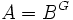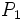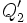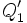# Going down for fixed-point subring under finite automorphism group

This fact is an application of the following pivotal fact/result/idea: going up theorem
View other applications of going up theorem OR Read a survey article on applying going up theorem

## Statement

Suppose$B$ is a commutative unital ring and$G$ is a finite subgroup of the automorphism group of$B$. Suppose$A = B^G$is the fixed-point subring. Then, the extension$B$ of$A$ is a going down extension; in other words, we have going down for prime ideals. Explicitly:

If$P_1 \supset P_2$ are prime ideals of$A$ and$Q_1$ is a prime ideal of$B$ contracting to$P_1$ then there exists a prime ideal$Q_2$ of$B$ contracting to$P_2$, such that$Q_2 \subset Q_1$.

## Definitions used

Fill this in later

## Proof

### Proof outline

• Show that$B$ is an integral extension of$A$
• Use the fact that integral extensions give surjective maps on spectra,to find a prime ideal$Q_2'$ lying over$P_2$
• Use going up to find a prime ideal$Q_1'$ lying over$P_1$ and containing$Q_2'$
• Use the transitivity of action of$G$ on the fiber over$P_1$ to find an automorphism that sends$Q_1'$ to$Q_1$
• The image of$Q_2'$ under that automorphism is the required$Q_2$